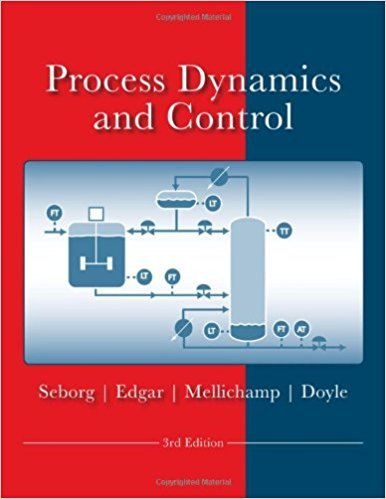×
Get Full Access to Process Dynamics And Control - 3 Edition - Chapter 4 - Problem 4.4
Get Full Access to Process Dynamics And Control - 3 Edition - Chapter 4 - Problem 4.4

×

# Consider the first-order transfer function model inISBN: 9780470128671 148

## Solution for problem 4.4 Chapter 4

Process Dynamics and Control | 3rd Edition

• Textbook Solutions
• 2901 Step-by-step solutions solved by professors and subject experts
• Get 24/7 help from StudySoup virtual teaching assistantsProcess Dynamics and Control | 3rd Edition

4 5 1 316 Reviews
15
2
Problem 4.4

Consider the first-order transfer function model in Exercise 4.2 where y and u are deviation variables. For an initial condition of y(O) = 1 and a step change in u of magnitude 2 (at t = 0), calculate the response, y(t). Hint: First determine the corresponding differential equation model by using the inverse Laplace tranform.

Step-by-Step Solution:
Step 1 of 3

CHE 481 Week 6 Notes Estimation of Capital Cost – Chapter 7 Economic Plant Design • PFD includes (stream table & equipment summary) • Next 4 chapters, use PFD for estimating : 1. Capital cost needed to build a new chemical plant 2. Operating cost needed to operate a chemical plant 3. Combine Capital & Operating cost to estimate profitability 4. Select “best process” from competing alternatives 5. Economics of change & modifications to existing plant 1 CLASSIFICATIONS OF CAPITAL COST ESTIMATES Five accepted classifications of capital cost estimates: 1) Order 0f Magnitude Estimate ( ratio or feasibility ) • Capital Cost from previous plants • Adjust for scale & inflation • Diagram : BFD • Least accurate + 40% , -20% 2. Study Estimate

Step 2 of 3

Step 3 of 3

##### ISBN: 9780470128671

Process Dynamics and Control was written by and is associated to the ISBN: 9780470128671. This textbook survival guide was created for the textbook: Process Dynamics and Control, edition: 3. Since the solution to 4.4 from 4 chapter was answered, more than 516 students have viewed the full step-by-step answer. The full step-by-step solution to problem: 4.4 from chapter: 4 was answered by , our top Engineering and Tech solution expert on 11/15/17, 04:03PM. The answer to “Consider the first-order transfer function model in Exercise 4.2 where y and u are deviation variables. For an initial condition of y(O) = 1 and a step change in u of magnitude 2 (at t = 0), calculate the response, y(t). Hint: First determine the corresponding differential equation model by using the inverse Laplace tranform.” is broken down into a number of easy to follow steps, and 55 words. This full solution covers the following key subjects: model, initial, condition, consider, corresponding. This expansive textbook survival guide covers 24 chapters, and 420 solutions.

Unlock Textbook Solution